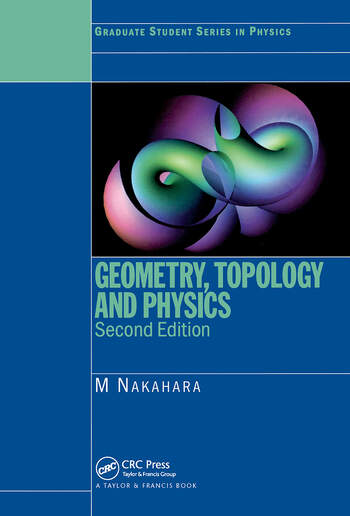# Geometry, Topology and Physics

## 2nd Edition

Mikio Nakahara

CRC Press
Published June 4, 2003
Textbook - 596 Pages
ISBN 9780750306065 - CAT# IP317

For Instructors Request Inspection Copy

USD\$94.95

FREE Standard Shipping!

## Preview

### Summary

Differential geometry and topology have become essential tools for many theoretical physicists. In particular, they are indispensable in theoretical studies of condensed matter physics, gravity, and particle physics. Geometry, Topology and Physics, Second Edition introduces the ideas and techniques of differential geometry and topology at a level suitable for postgraduate students and researchers in these fields.

The second edition of this popular and established text incorporates a number of changes designed to meet the needs of the reader and reflect the development of the subject. The book features a considerably expanded first chapter, reviewing aspects of path integral quantization and gauge theories. Chapter 2 introduces the mathematical concepts of maps, vector spaces, and topology. The following chapters focus on more elaborate concepts in geometry and topology and discuss the application of these concepts to liquid crystals, superfluid helium, general relativity, and bosonic string theory. Later chapters unify geometry and topology, exploring fiber bundles, characteristic classes, and index theorems. New to this second edition is the proof of the index theorem in terms of supersymmetric quantum mechanics. The final two chapters are devoted to the most fascinating applications of geometry and topology in contemporary physics, namely the study of anomalies in gauge field theories and the analysis of Polakov's bosonic string theory from the geometrical point of view.

Geometry, Topology and Physics, Second Edition is an ideal introduction to differential geometry and topology for postgraduate students and researchers in theoretical and mathematical physics.

#### Instructors

We provide complimentary e-inspection copies of primary textbooks to instructors considering our books for course adoption.